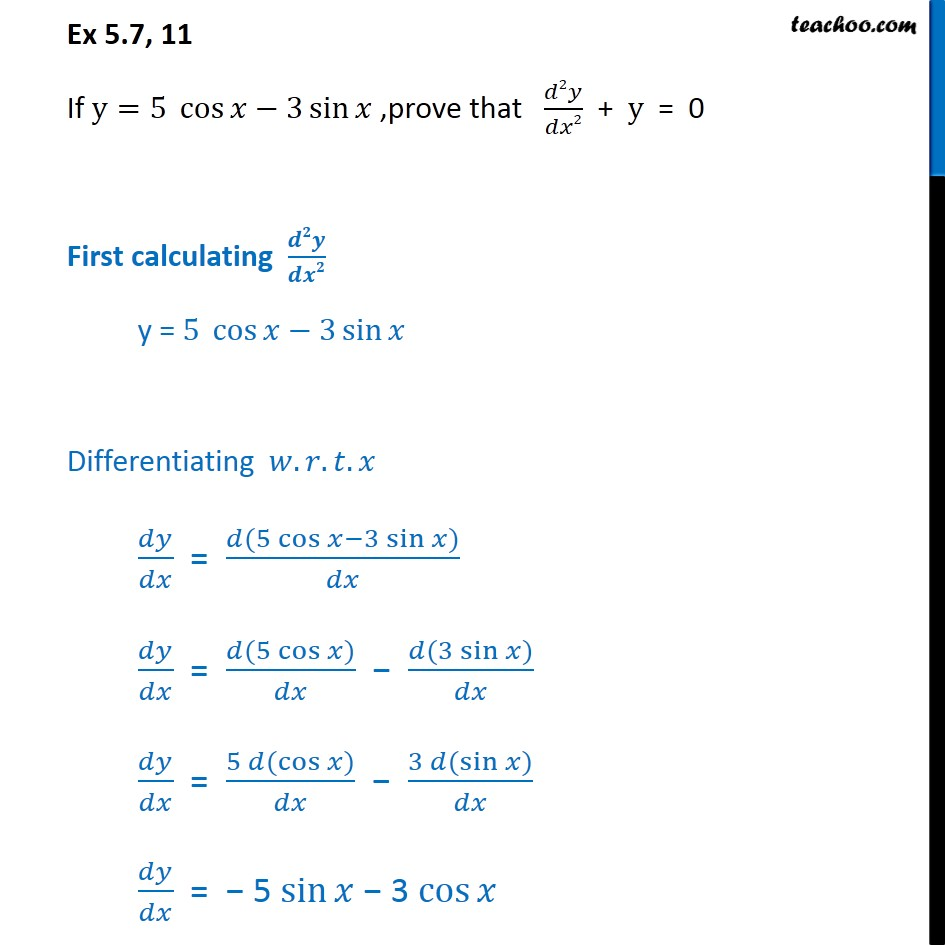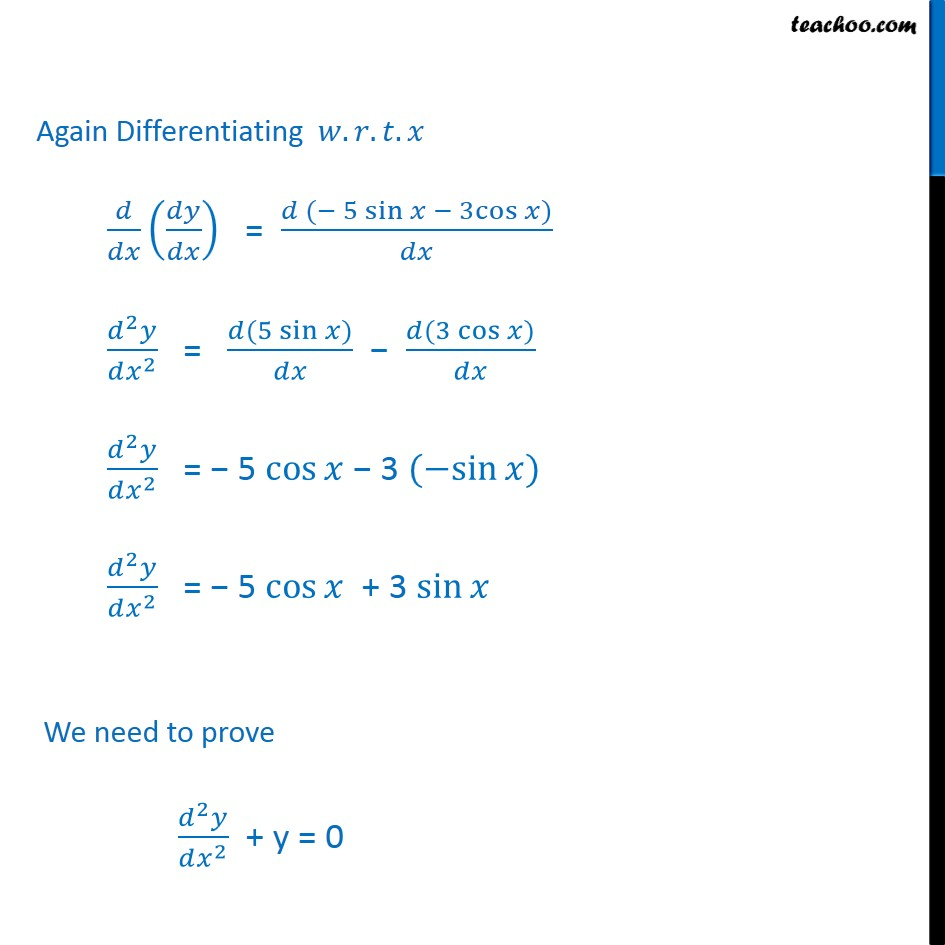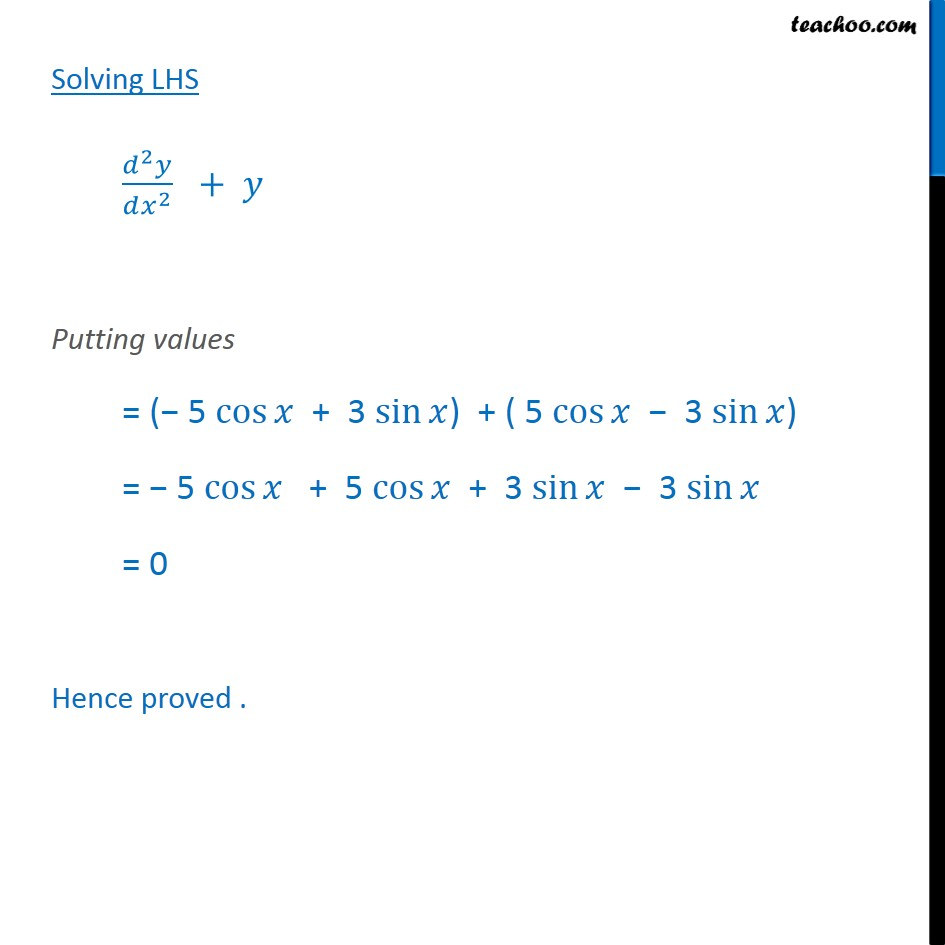1. Chapter 5 Class 12 Continuity and Differentiability
2. Serial order wise
3. Ex 5.7

Transcript

Ex 5.7, 11 If y=5 cos﷮𝑥−3 sin﷮𝑥﷯﷯ ,prove that 𝑑2𝑦﷮𝑑𝑥2﷯ + y = 0 First calculating 𝒅𝟐𝒚﷮𝒅𝒙𝟐﷯ y = 5 cos﷮𝑥−3 sin﷮𝑥﷯﷯ Differentiating 𝑤.𝑟.𝑡.𝑥 𝑑𝑦﷮𝑑𝑥﷯ = 𝑑(5 cos﷮𝑥−3 sin﷮𝑥﷯﷯)﷮𝑑𝑥﷯ 𝑑𝑦﷮𝑑𝑥﷯ = 𝑑(5 cos﷮𝑥﷯)﷮𝑑𝑥﷯ − 𝑑(3 sin﷮𝑥﷯)﷮𝑑𝑥﷯ 𝑑𝑦﷮𝑑𝑥﷯ = 5 𝑑( cos﷮𝑥﷯)﷮𝑑𝑥﷯ − 3 𝑑( sin﷮𝑥﷯)﷮𝑑𝑥﷯ 𝑑𝑦﷮𝑑𝑥﷯ = − 5 sin﷮𝑥﷯ − 3 cos﷮𝑥﷯ Again Differentiating 𝑤.𝑟.𝑡.𝑥 𝑑﷮𝑑𝑥﷯ 𝑑𝑦﷮𝑑𝑥﷯﷯ = 𝑑 (− 5 sin﷮𝑥﷯ − 3cos﷮𝑥)﷯﷮𝑑𝑥﷯ 𝑑﷮2﷯𝑦﷮𝑑 𝑥﷮2﷯﷯ = 𝑑(5 sin﷮𝑥﷯)﷮𝑑𝑥﷯ − 𝑑(−3 cos﷮𝑥﷯)﷮𝑑𝑥﷯ 𝑑﷮2﷯𝑦﷮𝑑 𝑥﷮2﷯﷯ = − 5 cos﷮𝑥﷯ − 3 (−sin﷮𝑥)﷯ 𝑑﷮2﷯𝑦﷮𝑑 𝑥﷮2﷯﷯ = − 5 cos﷮𝑥﷯ + 3 sin﷮𝑥﷯ We need to prove 𝑑﷮2﷯𝑦﷮𝑑 𝑥﷮2﷯﷯ + y = 0 Solving LHS 𝑑﷮2﷯𝑦﷮𝑑 𝑥﷮2﷯﷯ + 𝑦 Putting values = (− 5 cos﷮𝑥﷯ + 3 sin﷮𝑥﷯) + ( 5 cos﷮𝑥﷯ − 3 sin﷮𝑥﷯) = − 5 cos﷮𝑥﷯ + 5 cos﷮𝑥﷯ + 3 sin﷮𝑥﷯ − 3 sin﷮𝑥﷯ = 0 Hence proved .

Ex 5.7